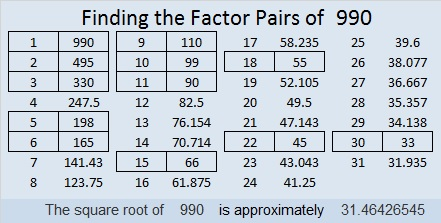# 990 Christmas Factor Trees

Contents

### Today’s Puzzle:

This puzzle has a couple of small Christmas trees in it. Don’t let their smallness fool you into thinking this is an easy puzzle. Can you solve it? Remember to use logic and not guess and check to find the solution.Print the puzzles or type the solution in this excel file: 10-factors-986-992

### Factors of 990:

• 990 is a composite number.
• Prime factorization: 990 = 2 × 3 × 3 × 5 × 11, which can be written 990 = 2 × 3² × 5 × 11
• The exponents in the prime factorization are 1, 2, 1, and 1. Adding one to each and multiplying we get (1 + 1)(2 + 1)(1 + 1)(1 + 1) = 2 × 3 × 2 × 2 = 24. Therefore 990 has exactly 24 factors.
• Factors of 990: 1, 2, 3, 5, 6, 9, 10, 11, 15, 18, 22, 30, 33, 45, 55, 66, 90, 99, 110, 165, 198, 330, 495, 990
• Factor pairs: 990 = 1 × 990, 2 × 495, 3 × 330, 5 × 198, 6 × 165, 9 × 110, 10 × 99, 11 × 90, 15 × 66, 18 × 55, 22 × 45, or 30 × 33
• Taking the factor pair with the largest square number factor, we get √990 = (√9)(√110) = 3√110 ≈ 31.464265### Sum-Difference Puzzle:

990 has twelve factor pairs. One of the factor pairs adds up to 101, and a different one subtracts to 101. If you can identify those factor pairs, then you can solve this puzzle!### More about the Number 990:

There are many interesting facts about the number 990:

9 × 10 × 11 = 990

Because 44 × 45/2 = 990, it is the 44th triangular number. That means that the sum of all the numbers from 1 to 44 is 990.

990 is the sum of the twelve prime numbers from 59 to 107.
It is also the sum of six consecutive prime numbers:
151 + 157 + 163 + 167 + 173 + 179 = 990,
and the sum of two consecutive primes:
491 + 499 = 990

990 is the hypotenuse of a Pythagorean triple:
594-792-990 which is (3-4-5) times 198

990 looks interesting in some other bases:
It is 6A6 in BASE 12 (A is 10 base 10) because 6(144) + 10(12) + 6(1) = 990,
2E2 in BASE 19 (E is 14 base 10) because 2(19²) + 14(19) + 2(1) = 990
1K1 in BASE 23 (K is 20 base 10) because 1(23²) + 20(23) + 1(1) = 990
UU in BASE 32 (U is 30 base 10) because 30(32) + 30(1) = 30(33) = 990
U0 in BASE 33 because 30(33) = 990

This site uses Akismet to reduce spam. Learn how your comment data is processed.